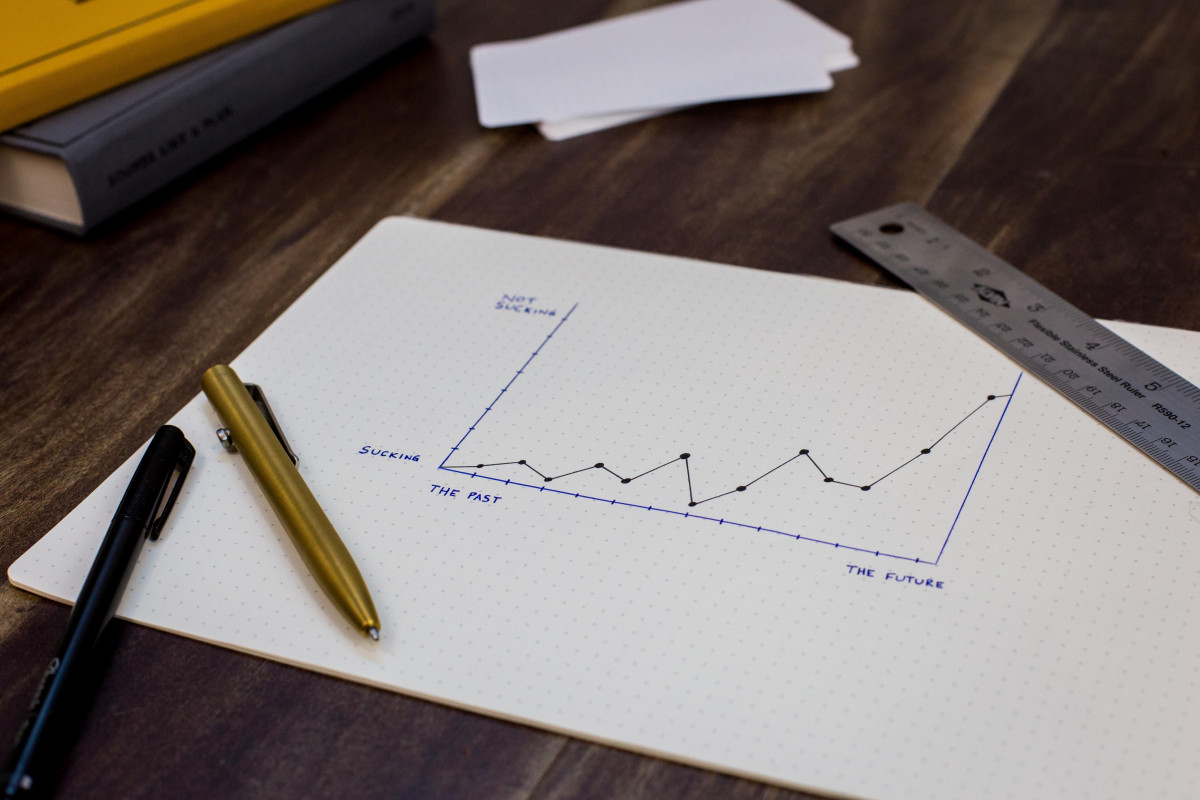# Tips on using Google Sheets for an old Excel and 1-2-3 deal# Tips on using Google Sheets for an old Excel and 1-2-3 deal

Google Sheets is wonderful for cooperative spreadsheet development, but really using it there a number of formulas tips and tricks:

1. Blank cells. If you want to detect if a cell has a number, then BLANK() and then if you want to see if a cell is non-blank, then NOT(BLANK())
2. Indexing in a range. If you have a table then you can use VLOOKUP() if the numbers are sorted, but if you want to figure out what is a particular row and column, the and that makes it easy.
3. Vlookup. This is perfect for looking numbers up in a table. Note that normally these won't be sorts, so make sure the third parameter is FALSE, so vlookup("hello",a1:b23,2, false) means look for the word hello in the lookup table at a1:b23 and return the value in the 2nd column and assume the list isn't sorted.
4. Regex. If you want to check is a string is one of several and then spit out a number, then regexmatch is your friend. So this would look like `Regexmatch(a1, "(office|work)*") which would match either of these strings that are in between the (parenthesis)
5. Roundup. If you normally just to INT(A1+0.5), then you will appreciate that there is actually a function that rounds up to any arbitrary digit, so ROUNDUP(23.234, -1) works and you get 23.2.
6. SUMIFS. This is a super useful function, you basically give it a range and it will pick out elements and sum them if a criterion is met. So you can, for instance, add up inventory based on a bunch of different conventions. It's a poor man's SQL Select statement. There is a simpler version called SUMIF that inverts the range and criteria so be careful.
7. TRIM. This is super useful if you have a bunch of white space around a header, it just cuts it all.